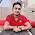### Armstrong Number Check/Generation - LISP

1.To Check

Program

(defun str(n)
(setf num n sum 0)
(loop while (/= n 0) do
(setf digit (mod n 10))
(setf sum (+ sum (* (* digit digit) digit)))
(setf n (floor n 10))
)
(if(= sum num) (print "Armstrong Number....")
(print "Not a Armstrong Number....")
)
t)

Output:

T
Break 20 > (str 153)

"Armstrong Number...."
T
Break 20 >

2.To Generate

Program:

(defun arm(rang)
(do((j 1 (+ j 1))) ((= j rang))
(setf n j)
(setf num n sum 1)
(loop for i from n above 0 do
(setf f1 (mod n 10))
(setf sum (+ sum (* (* f1 f1) f1)))
(setf n (floor n 10))
(setf i n)
)
(if(= sum num) (print num))
)
t
)

Output:

T
Break 15 > (str 157)

153
T
Break 15 >

1.Armstrong Program in Java

In general, Armstrong number is a number that is the sum of its own digits each raised to the power of the number of digits is equal to the number itself.
Simple example of armstrong number is 153, 1 ^ 3 + 5 ^ 3 + 3 ^ 3 = 153

2.3.After reading this web site I am very satisfied simply because this site is providing comprehensive knowledge for you to audience.
Thank you to the perform as well as discuss anything incredibly important in my opinion. We loose time waiting for your next article writing in addition to I beg one to get back to pay a visit to our website in

selenium training in Bangalore
selenium training in Marathahalli
selenium training in Btm layout
selenium training in Jaya nagar
selenium training in Electronic city
selenium training in Kalyan nagar